CBSE Class 10 Sample Paper for 2019 Boards

Class 10
Solutions of Sample Papers for Class 10 Boards

Question 27 (OR 2 nd   question)

The angle of elevation of a cloud from a point 60 m above the surface of the water of a lake is 30° and the angle of depression of its shadow from the same point in water of lake is 60° . Find the height of the cloud from the surface of water.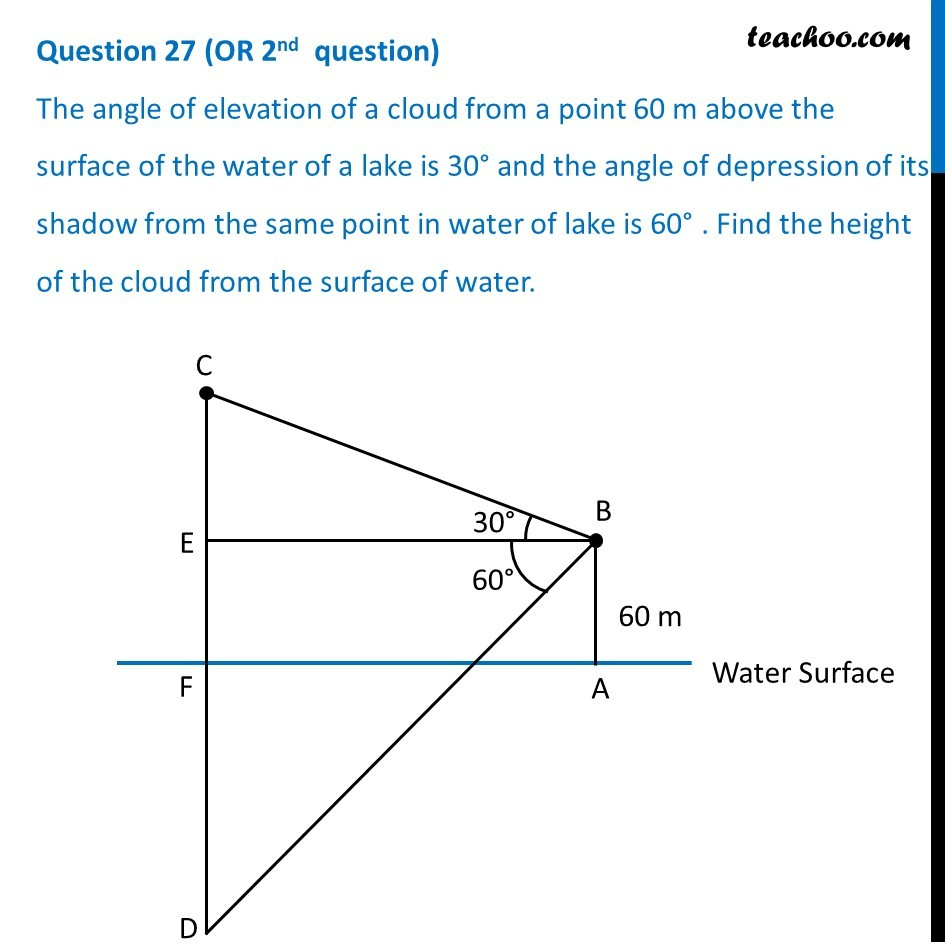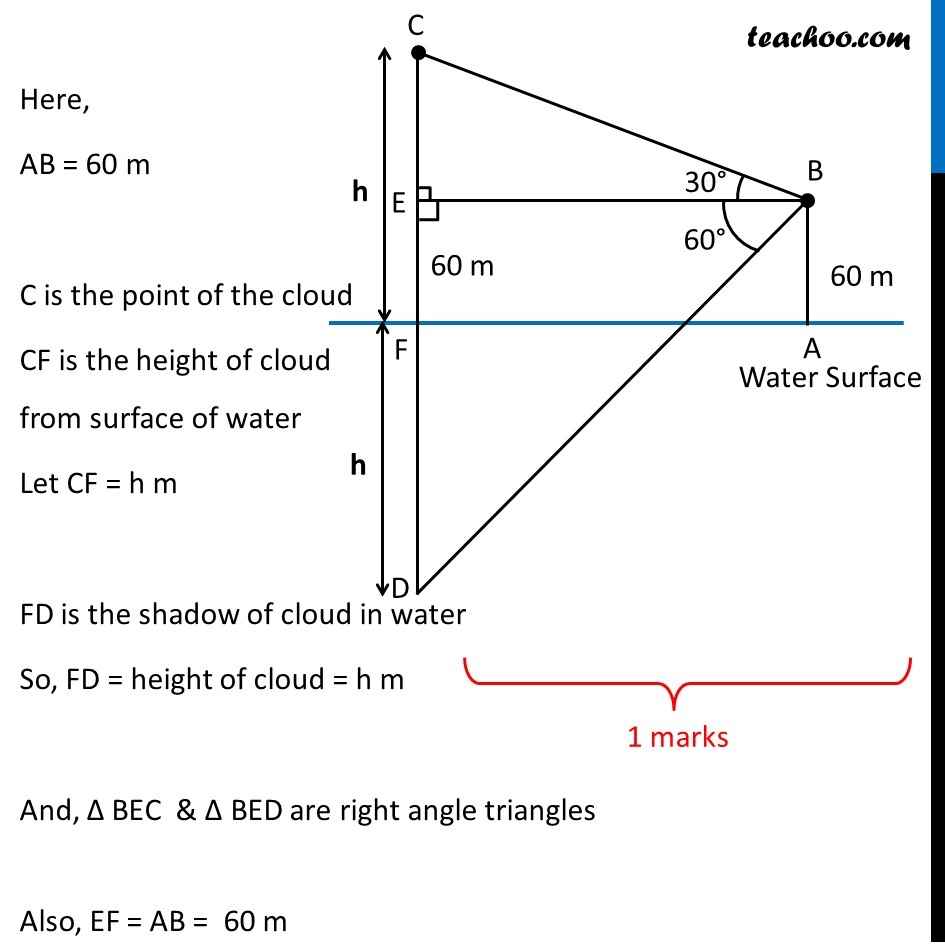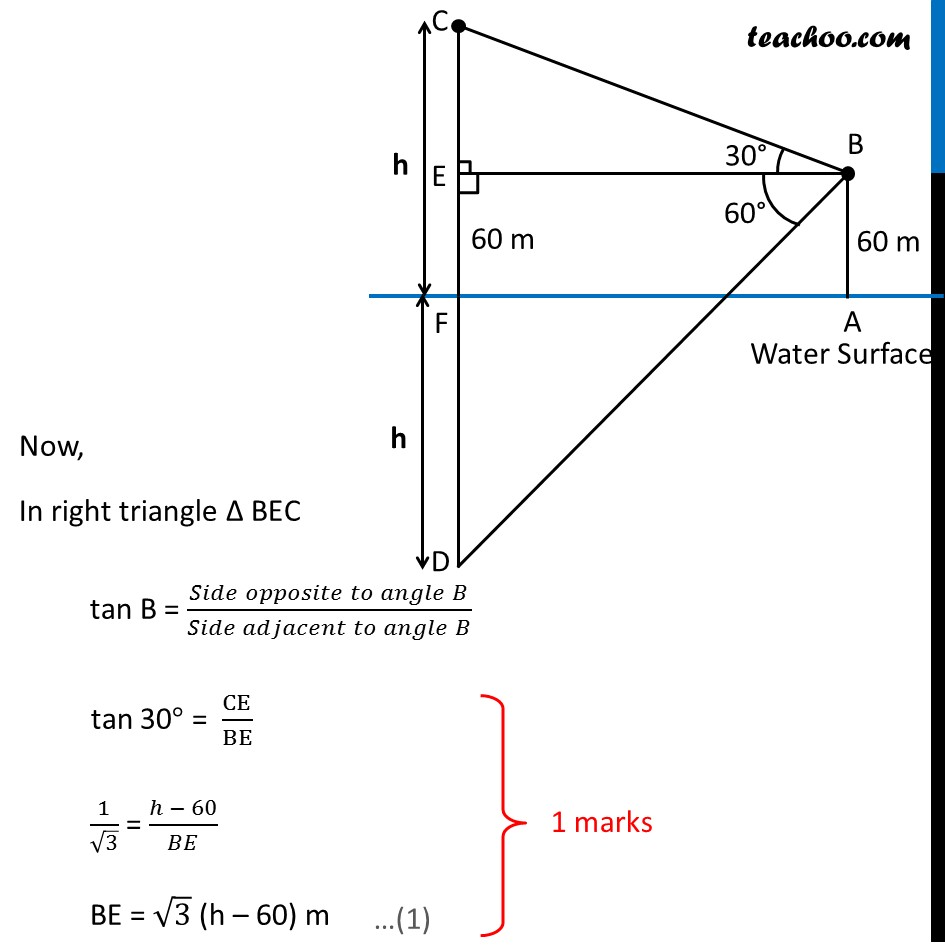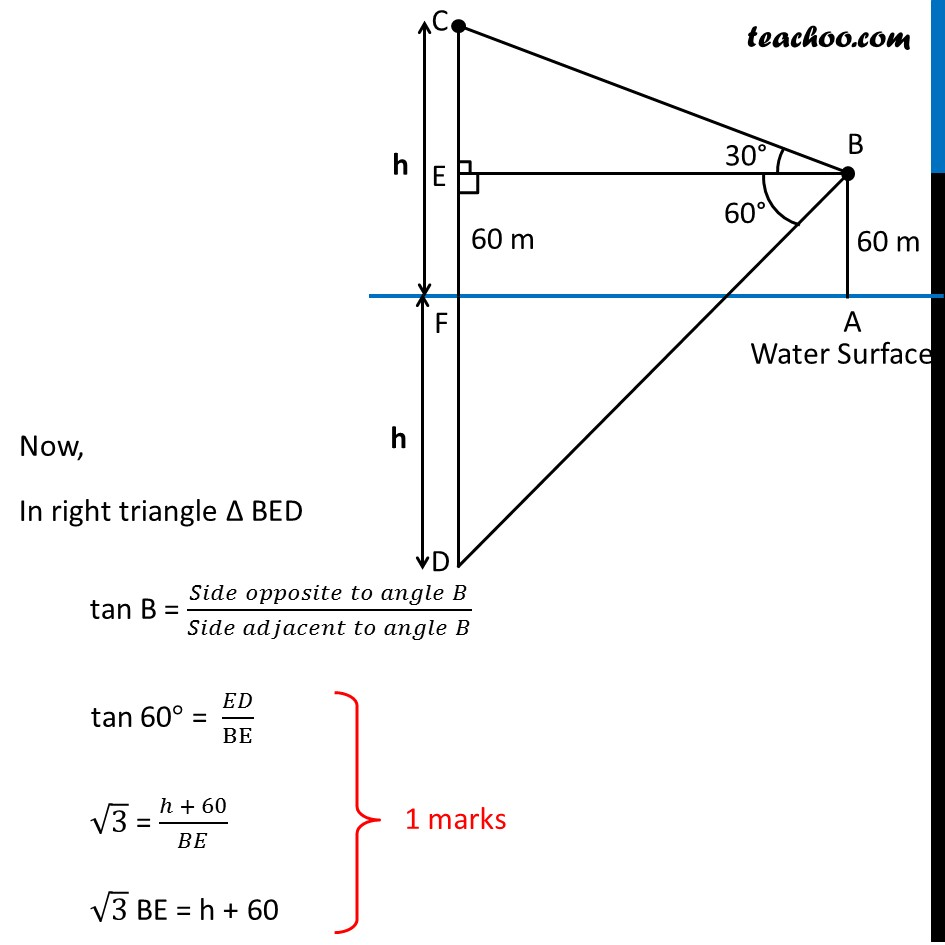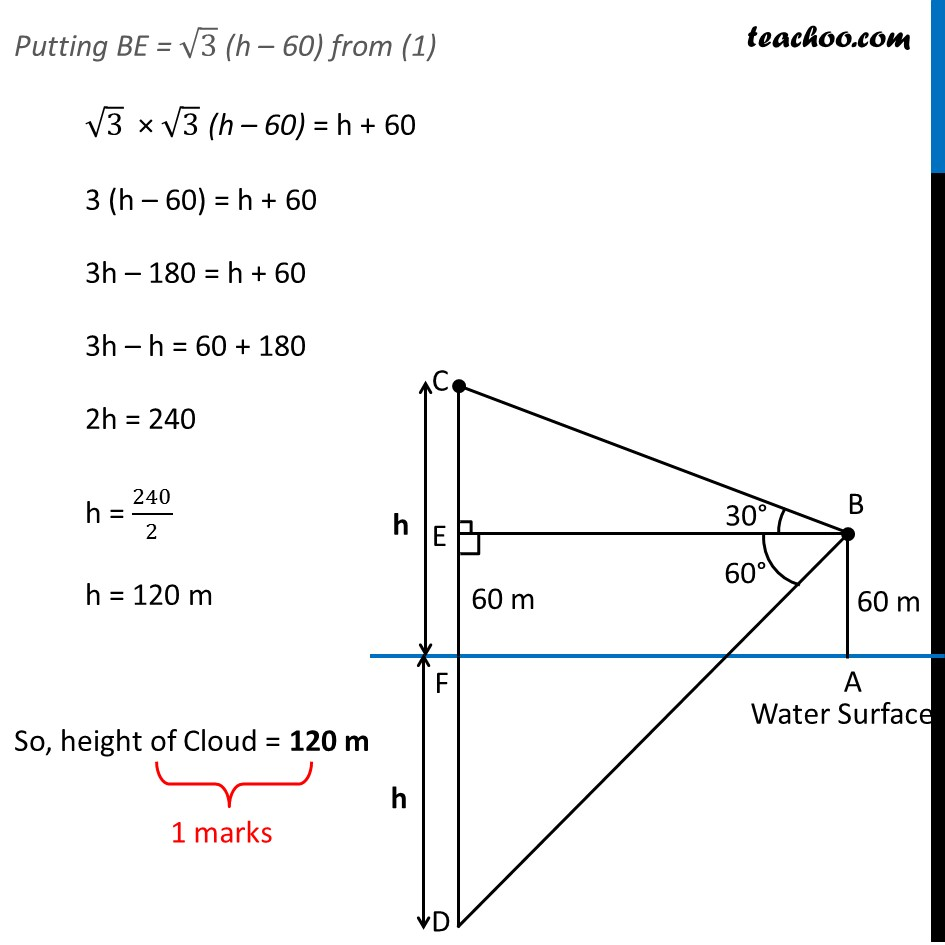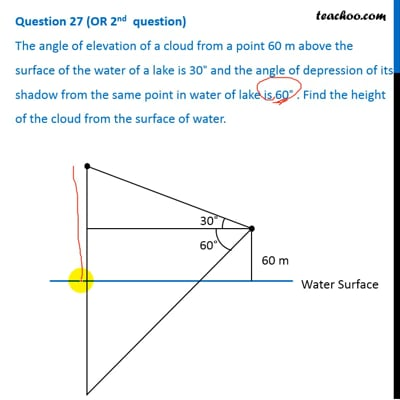This video is only available for Teachoo black users

### Transcript

Question 27 (OR 2nd question) The angle of elevation of a cloud from a point 60 m above the surface of the water of a lake is 30° and the angle of depression of its shadow from the same point in water of lake is 60° . Find the height of the cloud from the surface of water. Here, AB = 60 m C is the point of the cloud CF is the height of cloud from surface of water Let CF = h m FD is the shadow of cloud in water So, FD = height of cloud = h m And, Δ BEC & Δ BED are right angle triangles Also, EF = AB = 60 m Now, In right triangle Δ BEC tan B = (𝑆𝑖𝑑𝑒 𝑜𝑝𝑝𝑜𝑠𝑖𝑡𝑒 𝑡𝑜 𝑎𝑛𝑔𝑙𝑒" " 𝐵)/(𝑆𝑖𝑑𝑒 𝑎𝑑𝑗𝑎𝑐𝑒𝑛𝑡 𝑡𝑜 𝑎𝑛𝑔𝑙𝑒" " 𝐵) tan 30° = CE/BE 1/√3 = (ℎ − 60)/𝐵𝐸 BE = √3 (h – 60) m Now, In right triangle Δ BED tan B = (𝑆𝑖𝑑𝑒 𝑜𝑝𝑝𝑜𝑠𝑖𝑡𝑒 𝑡𝑜 𝑎𝑛𝑔𝑙𝑒" " 𝐵)/(𝑆𝑖𝑑𝑒 𝑎𝑑𝑗𝑎𝑐𝑒𝑛𝑡 𝑡𝑜 𝑎𝑛𝑔𝑙𝑒" " 𝐵) tan 60° = 𝐸𝐷/BE √3 = (ℎ + 60)/𝐵𝐸 √3 BE = h + 60 Putting BE = √3 (h – 60) from (1) √3 × √3 (h – 60) = h + 60 3 (h – 60) = h + 60 3h – 180 = h + 60 3h – h = 60 + 180 2h = 240 h = 240/2 h = 120 m So, height of Cloud = 120 m